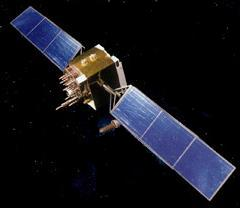### GPS

 The Global Positioning Satellite System is a space navigation system operated by the United States Air Force Space Command. It is available for military and civilian use, and at the present time is the most widely used navigation system in the world. A minimum of 24 satellites are maintained in near circular semisynchronous orbits spread over 6 different orbital planes.GPS TRANSMIT FREQUENCIES

 Channel Frequency/MHz Use Comments L1 1575.42 Civilian C/A and P codes L2 1227.60 Military P code L3 1381.05 NUDET Nuclear detonation detection L4 1842.40 Ionospheric Correction Minimal ionospheric delay L5 1176.45 Civilian Second civilian channel S1 2227.50 Telemetry Satellite systems monitoring

Notes:
1. Not all satellites use all the frequencies listed above. Early satellites in the series transmitted only the L1, L2 and L3 channels.
2. C/A stands for coarse acquisition code, P stands for precision code.
3. Information is impressed (modulated) on the respective carrier signals using spread spectrum techniques. Pseudo-random code sequences are used. The C/A (coarse/acquisition) modulation has a repetition length of 1023 bits, and the modulated bit rate is 1.023 million bits per second, thus repeating the code in one millisecond. The P (precision) modulation has a pseudo-random code length of six trillion (6 x 1012) bits and a modulation rate ten times that of the C/A code. The C/A pseudo-random sequence is widely available (clear access), whereas the P sequence is restricted to selected users. Superimposed on the C/A and P codes is a low data rate (50 bps) modulation which transmits information about the satellite ephemeris, clock and other corrections and information about the status of both the particular satellite and the GPS system.
4. GPS carrier frequencies are an integral multiple of the high speed (P code) chip rate:

L1 = 1.57542 GHz = 154 x 10.230
L2 = 1.22760 GHz = 120 x 10.230
5. The nuclear burst detection (NUDET) capability consists of three sensors: an optical sensor, an X-ray dosimeter and an EMP (electromagnetic pulse) detector. The presence of very accurate clocks on board the satellite enables time-of-flight measurements from different satellites to compute the position of the nuclear explosion. The L3 channel is encrypted.

Satellite Orbital Data:

• Orbital altitude = 20,200 km
• Orbital period = 718 minutes (11 hrs 58 m)
• Orbital inclination = 55 degrees
• Satellites are spaced in 6 orbital planes, 60 degrees apart
• Notes:

1. The orbital period is half the Earth's sidereal rotation rate, and thus the satellite has the same ground track every second orbit, although 4 minutes earlier (solar time) each day.
2. Some of the early satellites had an orbital inclination of 63 degrees.

Satellite Timing:

Current GPS satellites carry two rubidium and two cesium clocks. Timing accuracy is estimated at one second in 160,000 years. Despite this, timing corrections are uplinked daily.

### General Data

• Speed of light c = 299792.458 km s-1
• 1 nanosecond timing error = 0.300 metres range error>/li>

### Ionospheric Effects Data

The ionosphere is a plasma existing in the upper atmosphere between the approximate altitudes of 80 to 800 km. Radio waves passing through this plasma will be retarded (slowed down). The amount of retardation may be expressed in terms of time or equivalent range. It is proportional to the columnar electron content (electrons per square metre) of the ionosphere and the inverse square of the signal frequency. The total electron content [TEC] between the satellites and ground is typically between a few and several hundred TECU where 1 TEC Unit = 1016 electrons/m2. Specific timing and ranging errors for different TEC values are specified at the L1 GPS frequency in the table below.

TECURange Error(m)Time error(ns)
10.1630.542
101.635.42
10016.354.2